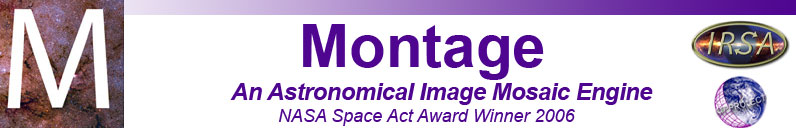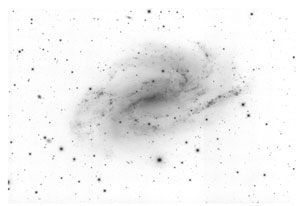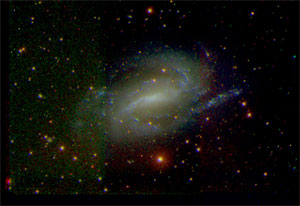# mJPEG

Description:
Generates a JPEG image file from a FITS file (or a set of three FITS files in color). A data range for each image can be defined, and the data can be stretched by any power of the log() function (including zero: linear). Pseudo-color color tables can be applied in single-image mode.
Note: If creating a true color image, all input images must have identical WCS information (ie, same size, resolution, coordinate system, etc).
Syntax:
mJPEG [-d] [-t power] [-s statusfile] [-ct color-table]
[-gray in.fits minrange maxrange [logpower/gaussian]
[-red red.fits rminrange rmaxrange [rlogpower/gaussian]
[-green green.fits gminrange gmaxrange [glogpower/gaussian]
[-blue blue.fits bminrange bmaxrange [blogpower/gaussian]
-out out.jpg

Switches:

-d
Print out additional debugging information
-t power
Increasing the value of power will enhance the contrast between colors; useful when all pixels are close to white, but you wish to accentuate the color difference.
-s statusfile
Write output and errors to statusfile instead of stdout
-ct color-table
Choose a color table for pseudo-color images; color-table can be an integer between 0 and 11. Click here for samples of each color table.

Arguments:

mJPEG takes as arguments a list of FITS images to be used as input for the true- or pseudo-color image. To create a pseudo-color image from a single FITS file, use the -gray flag; to create a true-color image, use the -red, -green, and -blue flags together. The syntax for each is the same:

-color
Provide the image name and stretch information to be used for color (one of: "gray", "red", "green", "blue")
color.fits
Filename of input image for this color. Can use a specific FITS extension by including it as: "filename.fits[X]", where X is the HDU number.
minrange maxrange
Lower/upper pixel values to use when stretching the image. Can also be expressed as a percentage, a sigma value, or any combination of these three. Some examples:
• 0 100
• 0s 99.999%
• -1s 1s
logpower/gaussian
Specify a log power to use in stretching the image. Can be a positive integer or one of:
• log
• loglog
• linear (default)
-out out.jpg
Path to the output JPEG image
Results:
The output message includes stretch information from the creation of the JPEG:
Psuedo-color (gray input only):
[struct stat="OK", min=datamin, minpercent=minfrac, max=datamax, maxpercent=maxfrac]
Three-color (red/green/blue inputs):
[struct stat="OK", bmin=bdatamin, bminpercent=bminfrac, bmax=bdatamax, bmaxpercent=bmaxfrac, gmin=gdatamin, gminpercent=gminfrac, gmax=gdatamax, gmaxpercent=gmaxfrac, rmin=rdatamin, rminpercent=rminfrac, rmax=rdatamax, rmaxpercent=rmaxfrac]

Examples:

To create a grayscale image from a FITS file:

\$ mJPEG -ct 1 -gray SINGS_B.fits 0s 99.999% gaussian -out SINGS_B.jpg
[struct stat="OK", min=0.391706, minpercent=50.00, max=136.555, maxpercent=100.00]

Output grayscale JPEG:To create a true 3-color image from three input images:

\$ mJPEG -t 2 -blue SINGS_B.fits 0s 99.999% gaussian
-green SINGS_R.fits 0s 99.999% gaussian
-red SINGS_I.fits 0s 99.999% gaussian
-out SINGS_3color.jpg
[struct stat="OK", bmin=0.391706, bminpercent=50.00, bmax=136.555, bmaxpercent=100.00, gmin=2.98851, gminpercent=50.00, gmax=366.148, gmaxpercent=100.00, rmin=4.26902, rminpercent=50.00, rmax=295.179, rmaxpercent=100.00]

Output 3-color JPEG:Return Codes:

• [struct stat="OK", min=datamin, minpercent=minfrac, max=datamax, maxpercent=maxfrac]
• [struct stat="OK", bmin=bdatamin, bminpercent=bminfrac, bmax=bdatamax, bmaxpercent=bmaxfrac, gmin=gdatamin, gminpercent=gminfrac, gmax=gdatamax, gmaxpercent=gmaxfrac, rmin=rdatamin, rminpercent=rminfrac, rmax=rdatamax, rmaxpercent=rmaxfrac]
• [struct stat="ERROR", msg="Usage: mJPEG [-d] [-t(rue-color) power] [-s statusfile] [-ct color-table] -gray in.fits minrange maxrange [logpower/gaussian] -red red.fits rminrange rmaxrange [rlogpower/gaussian] -green green.fits gminrange gmaxrange [glogpower/gaussian] -blue blue.fits bminrange bmaxrange [blogpower/gaussian] -out out.jpg"]
• [struct stat="ERROR", msg="Too few arguments following -ct flag"]
• [struct stat="ERROR", msg="Color table index must be between 0 and 11"]
• [struct stat="ERROR", msg="Too few arguments following -s flag"]
• [struct stat="ERROR", msg="Cannot open status file: statusfile"]
• [struct stat="ERROR", msg="Too few arguments following -color flag"]
• [struct stat="ERROR", msg="Image file in.fits invalid FITS"]
• [struct stat="ERROR", msg="Can't find HDU X"]
• [struct stat="ERROR", msg="Too few arguments following -out flag"]
• [struct stat="ERROR", msg="Error opening output file "out.jpg"]
• [struct stat="ERROR", msg="No input 'color' FITS file name given"] (in true-color mode)
• [struct stat="ERROR", msg="No input FITS file name given"] (in pseudo-color mode)
• [struct stat="ERROR", msg="No input JPEG file name given"]
• [struct stat="ERROR", msg="Color mode but no color image given"]
• [struct stat="ERROR", msg="WCS init failed for [fitsfile]"]
• [struct stat="ERROR", msg="Red and green FITS images don't match."]
• [struct stat="ERROR", msg="Red and blue FITS images don't match."]
• [struct stat="ERROR", msg="Grayscale/pseudocolor mode but no gray image given"]
• [struct stat="ERROR", msg="min percentile value "minrange%" is not a real number"]
• [struct stat="ERROR", msg="min sigma value "minranges" is not a real number"]
• [struct stat="ERROR", msg="min value "minrange" is not a real number"]
• [struct stat="ERROR", msg="max percentile value "maxrange%" is not a real number"]
• [struct stat="ERROR", msg="max sigma value "maxranges" is not a real number"]
• [struct stat="ERROR", msg="max value "maxrange" is not a real number"]
• [struct stat="ERROR", msg="beta percentile value "betaval%" is not a real number"]
• [struct stat="ERROR", msg="beta sigma value "betavals" is not a real number"]
• [struct stat="ERROR", msg="beta value "betaval" is not a real number"]
• [struct stat="ERROR", flag=errcode, msg="FITS library error"]